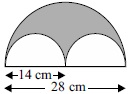# User ForumSubject :IMO    Class : Class 7

In the given figure, the area of the shaded portion isA308 cm2
B462 cm2
C154 cm2
D150 cm2

## Ans 1:

Class : Class 10
Option C is the answer

Class : Class 8
C is correct

Class : Class 6

## Ans 4:

Class : Class 8
c is correct308-154=154 you have done this308 154=462hence option c is correct

Class : Class 9
C is correct

Class : Class 8
c is correct

Class : Class 8
c is correct

## Ans 8:

Class : Class 8
Here the given answer option D is wrong . The correct answer is option C.

Class : Class 8
c is correct

## Ans 10:

Class : Class 8
Answer is (C)Diameter of bigger circle=28 cmradius=28/2=14 cmArea of semicircle=1/2*22/7*14*14=4312/14=308 sq.cm2 semicircle=circleArea=22/7*7*7=1078/7=154 sq.cmArea left=308-154=154 cm

## Ans 11:

Class : Class 4
option C,154 is correct answer

Class : Class 9

Class : Class 9
c is correct

Class : Class 8

Class : Class 8
C for corecct

Class : Class 8

## Ans 17:

Class : Class 5
option third is right

Class : Class 10

## Ans 19:

Class : Class 8
Yes , i agree ...Option c is the ans.

## Ans 20:

Class : Class 7
C is the correct answer.